# Exercises: Chapter 5, Section 5

1. Generalize the divergence theorem to the case of an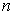-manifold with boundary in.

The generalization: Let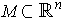be a compact-dimensional manifold-with-boundary andthe unit outward normal on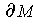. Let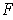be a differentiable vector fieldd on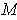. Then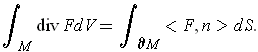As in the proof of the divergence theorem, let. Then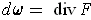. By Problem 5-25, on, we have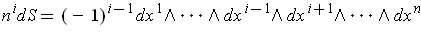for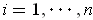. So,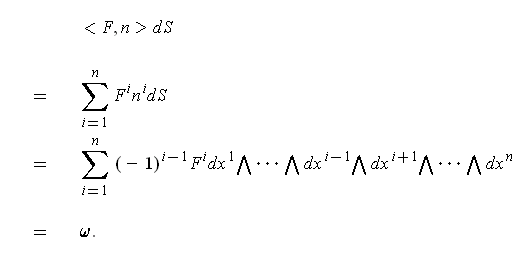By Stokes' Theorem, it follows that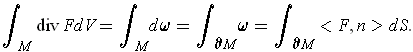2. Applying the generalized divergence theorem to the set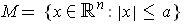and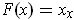, find the volume of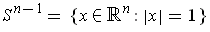in terms of the-dimensional volume of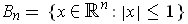. (This volume is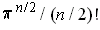ifis even and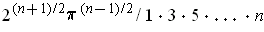ifis odd.)

One has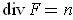and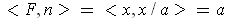since the outward normal is in the radial direction. So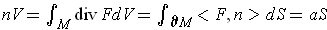. In particular, if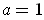, this says the surface area of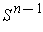istimes the volume of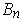.

3. Defineon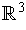by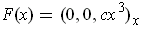and letbe a compact three-dimensional manifold-with-boundary with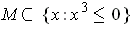. The vector fieldmay be thought of as the downward pressure of a fluid of density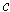in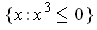. Since a fluid exerts equal pressures in all directions, we define the buoyaant force on, due to the fluid, as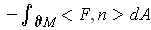. Prove the following theorem.

Theorem (Archimedes). The buoyant force onis equal to the weight of the fluid displaced by.

The definition of buoyant force is off by a sign.

The divergence theorem gives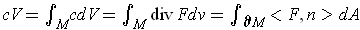. Now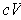is the weight of the fluid displaced by. So the right hand side should be the buoyant force. So one has the result if we define the buoyant force to be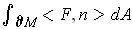. (This would make sense otherwise the buoyant force would be negative.)